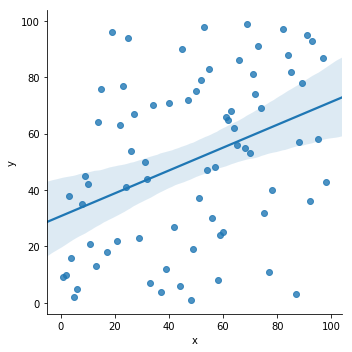How to determine Pearsons correlation in Python?
0

# How to determine Pearsons correlation in Python?

This recipe helps you determine Pearsons correlation in Python
In :
```def Snippet_120():
print()
print(format('How to determine Pearson\'s correlation in Python','*^82'))

import warnings
warnings.filterwarnings("ignore")

import matplotlib.pyplot as plt
import statistics as stats
import pandas as pd
import random
import seaborn as sns

# Create empty dataframe
df = pd.DataFrame()
df['x'] = random.sample(range(1, 100), 75)
df['y'] = random.sample(range(1, 100), 75)

# View first few rows of data

# Calculate Pearson’s Correlation Coefficient
def pearson(x,y):
# Create n, the number of observations in the data
n = len(x)
# Create lists to store the standard scores
standard_score_x = []; standard_score_y = [];
# Calculate the mean of x
mean_x = stats.mean(x)
# Calculate the standard deviation of x
standard_deviation_x = stats.stdev(x)
# Calculate the mean of y
mean_y = stats.mean(y)
# Calculate the standard deviation of y
standard_deviation_y = stats.stdev(y)
# For each observation in x
for observation in x:
# Calculate the standard score of x
standard_score_x.append((observation - mean_x)/standard_deviation_x)
# For each observation in y
for observation in y:
# Calculate the standard score of y
standard_score_y.append((observation - mean_y)/standard_deviation_y)
# Multiple the standard scores together, sum them, then divide by n-1, return that value
return (sum([i*j for i,j in zip(standard_score_x, standard_score_y)]))/(n-1)

# Show Pearson's Correlation Coefficient
result = pearson(df.x, df.y)
print()
print("Pearson\'s correlation coefficient is: ", result)
sns.lmplot('x', 'y', data=df, fit_reg=True)
plt.show()

Snippet_120()
```
```*****************How to determine Pearson's correlation in Python*****************

x   y
0  69  99
1  56  30
2  64  62
3  58   8
4  14  64

Pearson's correlation coefficient is:  0.3810462941506265
```In [ ]:
```
```